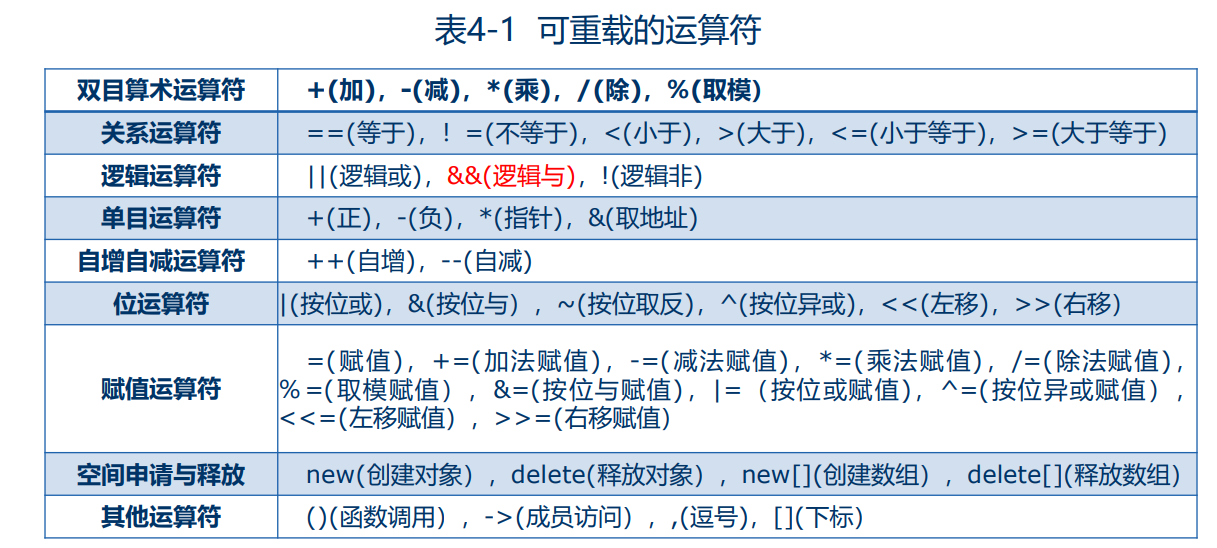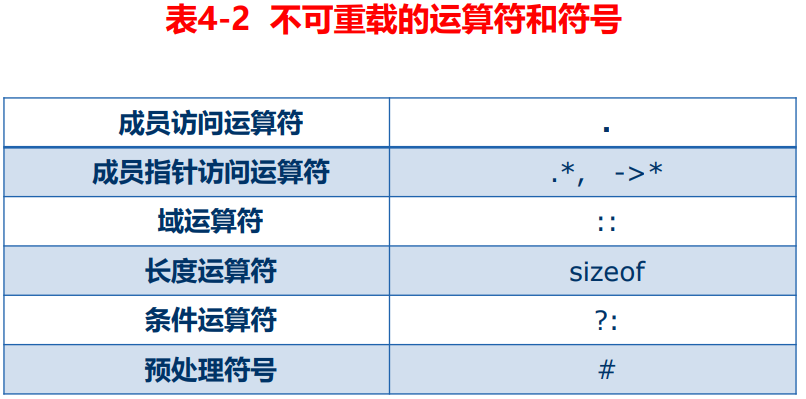# 导航

• 随笔 - 51
• 文章 - 0
• 评论 - 5
• 引用 - 0

## 一、运算符重载的概念

⚫ C++中的表达式由运算符和操作数按照规则构成。例如，算术运算符包括加“+”、减“-” 、乘“*” 、除“/”和取模“％”。如果不做特殊处理，则这些算术运算符通常只能用于对基本数据类型的常量或变量进行运算，而不能用于对象之间的运算。

⚫ 用于类运算的运算符通常都要重载。有两个运算符，系统提供了默认的重载版本：赋值运算符=地址运算符&### 2、重载运算符的规则

• 1)重载后运算符的含义应该符合原有的用法习惯。例如，重载“+”运算符，完成的功能就应该类似于做加法，在重载的“+”运算符中做减法是不合适的。
• 2)运算符重载不能改变运算符原有的语义，包括运算符的优先级和结合性。
• 3)运算符重载不能改变运算符操作数的个数及语法结构。
• 4)不能创建新的运算符，即重载运算符不能超出C++语言允许重载的运算符范围。
• 5)重载运算符“( )”“[ ]”“->”或者赋值运算符“=”时，只能将它们重载为成员函数，不能重载为全局函数。
• 6)运算符重载不能改变该运算符用于基本数据类型对象的含义。

3、赋值运算符的重载

• C++中的赋值运算符“=”要求左右两个操作数的类型是匹配的，或至少是赋值兼容的。有时希望“=”两边的操作数的类型即使不赋值兼容也能够成立，这就需要对“=”进行重载。C++规定，“=”只能重载为成员函数。若有类CL的两个对象s1和s2，则它们之间的赋值语句通常是下面这样的形式：s1=s2;当类CL中定义了成员函数，重载了赋值运算符后，上述赋值语句将解释为函数调用的形式：s1.operator=(s2);

4、浅拷贝和深拷贝

• 同类对象之间可以通过赋值运算符“=”互相赋值。如果没有经过重载，“=”的作用就是将赋值号右侧对象的值一一赋值给左侧的对象。这相当于值的拷贝，称为“浅拷贝”。
• 重载赋值运算符后，赋值语句的功能是将一个对象中指针成员变量指向的内容复制到另一个对象中指针成员变量指向的地方，这样的拷贝叫“深拷贝”

5、重载流插入运算符和流提取运算符

• 在C++中，左移运算符“<<”可以和cout一起用于输出，故常被称为“流插入运算符”。右移运算符“>>”和cin—起用于输入，一般被称为流提取运算符。它们都是C++类库中提供的。在类库提供的头文件中已经对“<<”和“>>”进行了重载，使之分别作为流插入运算符和流提取运算符，能用来输出和输入C++基本数据类型的数据。cout是ostream类的对象，cin是istream类的对象，它们都是在头文件iostream中声明的。因此，凡是用“cout<<”和“cin>>”对基本数据类型数据进行输出/输入的，都要用#include指令把头文件iostream包含到本程序文件中。
• 必须重载为类的友元
#include<iostream>
#include<typeinfo>
using namespace std;
class Point
{
private:
int x;
public:
Point(int x1){x=x1;}
int get();
Point operator++();
Point operator++(int x);
Point operator--();
Point operator--(int x);
void operator+(const Point &p);
void operator-(const Point &p);
Point& operator=(const Point &p);
operator double();
friend void operator<<(ostream & stream,Point obj);
};
int Point::get(){
return this->x;
}
//重载运算符（++obj）
Point Point::operator++(){
x++;
return *this;
}
//重载运算符（obj++）
Point Point::operator++(int x){
Point temp = *this;
this->x++;
return temp;
}
//重载运算符（--obj）
Point Point::operator--(){
this->x--;
return *this;
}
//重载运算符（obj--）
Point Point::operator--(int x){
Point temp = *this;
this->x--;
return temp;
}
//重载运算符+（a+b）
void Point::operator+(const Point &p){
this->x=this->x+p.x;
}
//重载运算符-（a-b）
void Point::operator-(const Point &p){
this->x=this->x-p.x;
}
//复制运算符重载=（a=b），赋值运算符必须重载为成员函数
Point& Point::operator=(const Point &p){
this->x=p.x;
return *this;
}
//重载类型转换运算符()a
Point::operator double(){
cout << "重载类型转换运算符" << endl;
return this->x;
}
//重载运算符 (cout <<)
void operator<<(ostream & stream,Point obj){
stream<< obj.x <<endl;
}

int main(){
Point point(10);
operator<<(cout, point.operator++());//11
Point point2(10);
operator<<(cout, point2.operator++(0));//10

Point point3(10);
operator<<(cout, point3.operator--());//9
Point point4(10);
operator<<(cout, point4.operator--(0));//10

Point point5(1);
Point point6(1);
point5.operator+(point6);
operator<<(cout, point5);//2

point5.operator-(point6);
operator<<(cout, point5);//1

Point point7(1);
Point point8(5);
operator<<(cout, point7.operator=(point8));//5

Point point9(20);
cout << typeid(point9.get()).name()<< endl;//i
cout << point9.operator double()<< endl;
cout << typeid(point9.operator double()).name();//d
return 0;
}

posted on 2020-06-06 11:13  君子生非异也  阅读(44)  评论(0编辑  收藏